• 21
•
• 4
•
• 3
•
•
•
28
Shares

# Differentiability

The concept of the differentiability (derivability) of a function tells us about whether a given function will be differentiable or not. If the function is differentiable then we will use Methods of differentiation to differentiate a function. Actually, this concept explains what it’s meant by the derivative (differentiation) of a function. Perquisites for learning this concept are the theory of Limits and the theory of Continuity. this whole concept is used to study functional equations.

## Meaning of Differentiability/Derivability

the phrase “Derivative/Differentiation of a function f(x) with respect to x” is denoted as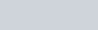it has two basic meanings-

#### 1. Geometrical Meaning

Geometrically derivative of a function f(x) at a point x=a means the slope of the tangent at x=a.

 It can be seen from the figure below that Tangent is a limiting case of a  Secant, and we calculate the slope of the tangent by finding the limiting value of the slope of a secant, This slope of the tangent is actually the Differentiation/derivative of a function at a point.as both two points approach closer the secant becomes a tangent.

Hence we concluded that the Derivative of a function simply means the slope of the tangent, Mathematically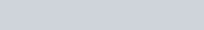##### Calculation of slope of tangent/First PrincipleSlope of secant shown is above figure is

(1)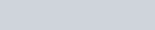As points, A and B approaches closer i.e. the horizontal separation h will tend to be negligible and the secant will become a tangent. Hence slope of the tangent will be equal to the limiting value of the slope of secant when the separation between A and B is negligible.

(2)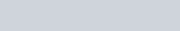The slope of the tangent is given by equation (2) is referred to as the First Principle of Differentiation by definition we know that this slope is derivative/differentiation of function.

#### 2. Physical Meaning

The Derivative/Differentiation of a function f(x) with respect to x at x=a, Physically means as the instantaneous rate of change of f(x) w.r.t x. Hence the slope at a given point on a graph gives an instantaneous rate of change. This physical interpretation of finding instantaneous rate of change is widely used in Various Disciplines whether it’s Physics, Chemistry, Accountancy, etc. for example- in physics, we find Instantaneous Velocity by differentiating displacement w.r.t time.

## Differentiability of a function at a Point

Any function is said to be Differentiable/Derivable at a point if there exists a Unique Tangent at that point whose slope is Finite. Also we Know that slope of tangent is expressed asFor the slope of the tangent to be finite, the above Limit should exist, For the Limit to exist The Left-Hand Limit (L.H.L.) and Right-Hand Limit (R.H.L) Both should exist and should be equal.
Hence the condition for the function to be differentiable at a point x=a is as following-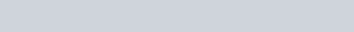It Should be noted that here the left-hand limit and right-hand limit are Referred to as The Left Hand Derivative and Right Hand Derivative Respectively.

## Differentiability of a function on an interval

### Differentiability in an Open Interval

Any function f(x) is Defined to be Differentiable/derivable over an interval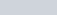if the function is differentiable at each and every point in the interval.
Note– it’s not feasible to check differentiability at each point since between any two points on a real line there are infinitely many points, Hence it is advised that One Should at least check at the critical points where the function changes its definition.

### Differentiability in a Closed Interval

Any function f(x) is Defined to be Differentiable/derivable over an interval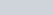if All the Following Conditions are Satisfied-
• The function Should be Differentiable/derivable over an interval• At end Points “a” and “b” the Right Hand Derivative and Left Hand Derivative Respectively should be finite.

## Theorems on Differentiability

If a Function is differentiable then it must be continuous but if the function is continuous this doesn’t mean that the function should be differentiable. Further, if the function is non-differentiable this doesn’t imply that function is discontinuous but if the function is discontinuous then it will be non-differentiable.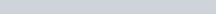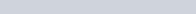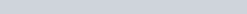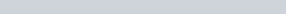the above can be visualized by analyzing the graph of modulus function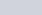at x=0, we can see that if we approach from the left-hand side and the right-hand side about x=0, there will be different tangents, since we don’t get unique tangent, the function is non-differentiable, but it’s continuous as we draw its graph without lifting the pen from the paper.

## Reasons For Non Differentiability

Any Non-differentiable/non-derivable function will be due to any of the following reasons-

#### Sharp Corners

At sharp corners in the graph of the function, the value of the left-hand derivative won’t be equal to the value of the right-hand derivative, in simple terms if we approach the graph of a function near sharp corner from the left side and right side we will not obtain a unique tangent, hence function will be non-drivable/differentiable.

#### Discontinuity

if the function is discontinuous then there will not be any unique tangent, hence the function will definitely be Non-differentiable.#### Vertical tangentif at a particular point the tangent to the curve of the function is perpendicular that is its slope will be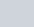, means the slope will not be finite hence, the function will be non-differentiable because for the function to be a differentiable unique tangent of finite slope should exist as defined earlier.

## Some important results on differentiability

 f(x) g(x) f(x)+g(x)          or f(x)-g(x) f(x).g(x)         or f(x)/g(x) Derivable Derivable Derivable Derivable Derivable Non Derivable Non Derivable May be Derivable Non Derivable Non Derivable May be Derivable May be Derivable
1. Every polynomial function, exponential function, and constant function is differentiable at each point of the real lime.
2. Logarithmic functions, trigonometric functions, and inverse trigonometric functions are differentiable in their domain.
3. The sum, differences, product, and quotient of two differentiable functions are differentiable.
4. The composition of a differentiable function is a differentiable function.
5. If a function is not differentiable but is continuous at a point, then geometrically implies that there is a sharp corner or any kink at the point.
6. If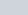and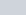both are not differentiable at a point, then the sum function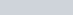and the product functioncan still be differentiable at a point.

### Tricks for problem-solving

• If a functionis differentiable at a pointthen it is a continuous function at.
• Ifis only continuous at point, then there is no guarantee thatis differentiable there.
• Ifis not differentiable at pointthen it may or may not be continuous at.
• Ifis not continuous at point, then it is not differentiable at.
• If left-hand derivative and right-hand derivative ofatare finite (they may or may not be equal), thenis continuous at.